# How To Convert A Human To Waves By Magick Package

ggplot2
magick
Author

Hiroaki Yutani

Published

November 23, 2018

I saw this tweet about Mathematica last year, which naturally urged me to write the R version of this code.

At that time, I faild because I didn’t know how to zoom images. But, now I know magick package. Let’s try again…

## Zoom images by magick

We can enlarge a image by either `image_resize()`, `image_scale()`, or `image_sample()`. I don’t know about the details of the differences, but it seems `image_resize()` does better for my purpose.

``library(magick)``
``````Linking to ImageMagick 6.9.12.3
Enabled features: cairo, freetype, fftw, ghostscript, heic, lcms, pango, raw, rsvg, webp
Disabled features: fontconfig, x11``````
``````rose <- image_convert(image_read("rose:"), "png")

roses <- c(
image_resize(rose, "400x"),
image_scale(rose, "400x"),
image_sample(rose, "400x")
)

image_append(roses, stack = TRUE)``````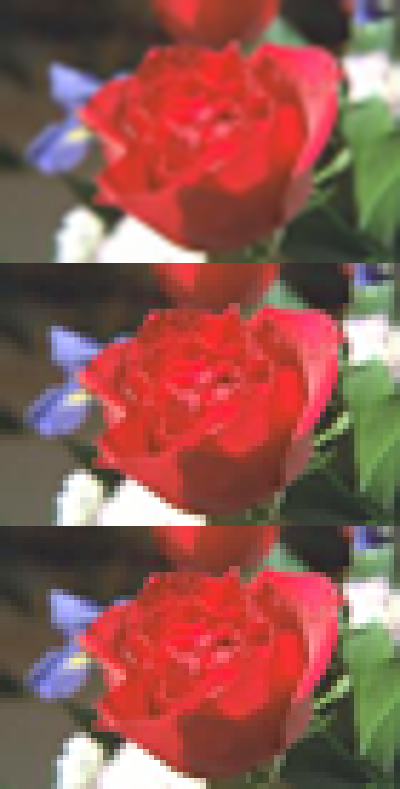But, zooming is not just about resizing; I want to focus on the center of the image as well. To do this, we can use `image_crop()`. But, it’s our job to calculate the perper offset to centering the image (IIUC, there’s no equivalent of `-gravity center` in magick package, right??).

Suppose we want to zoom by 200%. First of all, extract the original width and height.

``````info <- image_info(rose)
orig_width <- info\$width
orig_height <- info\$height``````

Then, let’s calculate the offset; to center the image, the offset should be half of the diffrence between the original size and the size you want.

``````width <- as.integer(orig_width * 2)
height <- as.integer(orig_height * 2)

offset_x <- as.integer((width - orig_width) / 2)
offset_y <- as.integer((height - orig_height) / 2)``````

Now we have enough information to crop the image. To provide these to Imagemagick, we need to learn a bit about Geometry syntax. It’s as simple as:

``<width>x<height>{+-}<xoffset>{+-}<yoffset>``

We can construct the geometries by `sprintf()` (use `%+d` instead of `%d` when we need explicit plus sign) as follows:

``(g_resize <- sprintf("%dx%d", width, height))``
`` "140x92"``
``(g_crop <- sprintf("%dx%d%+d%+d", orig_width, orig_height, offset_x, offset_y))``
`` "70x46+35+23"``

Now we can zoom

``````rose_zoomed <- rose %>%
image_resize(g_resize) %>%
image_crop(g_crop)

image_append(c(rose, rose_zoomed), stack = TRUE)``````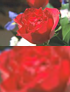## Zoom animatedly

Now that we know how to zoom, we can create a function to draw rose at the specified zoom level.

``````zoom_rose <- function(zoom = 1) {
width <- as.integer(orig_width * zoom)
height <- as.integer(orig_height * zoom)

offset_x <- as.integer((width - orig_width) / 2)
offset_y <- as.integer((height - orig_height) / 2)

g_resize <- sprintf("%dx%d", width, height)
g_crop <- sprintf("%dx%d%+d%+d", orig_width, orig_height, offset_x, offset_y)

rose %>%
image_resize(g_resize) %>%
image_crop(g_crop)
}

zoom_rose(1)``````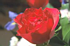``zoom_rose(2)``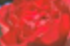The function can be applied to the vector of zoom levels by `lapply()`. Note that, to make the zoom speed looks constant, we need to power the steps.

``````steps <- 100
zooms <- 1 + 9 * (0:steps / steps)^2
imgs <- lapply(zooms, zoom_rose)``````

The list of images can be combined by `image_join()` and then can be converted to an animation by `image_animate()`

``````imgs %>%
image_join() %>%
image_animate(fps = 50)``````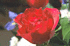## Result

Aparently, there are a lot of things to explain (expecially about involute of a circle), but it would be a bit too long… Let’s jump to the final version of my code and the result :P

``````library(ggplot2)
library(tibble)
library(dplyr, warn.conflicts = FALSE)

# the speed of involute increases uniformly; in order to make the lengths between
# steps equal, we need to calculate the square root
resolution_of_involute <- 10000L
t <- sqrt(seq(0, resolution_of_involute) / resolution_of_involute)

coil_turns <- 17L
max_r <- coil_turns * 2 * pi

# waviness of the coil
wave_frequency <- 100
wave_height_base <- pi

img_file <- tempfile(fileext = ".png")
download.file("https://hoxo-m.com/img/team/makiyama.png", destfile = img_file, mode = "wb")

# convert to grayscale
img_bw <- img_orig %>%
image_convert(type = "grayscale") %>%
image_modulate(brightness = 160) %>%
image_contrast(10)

# the width and height of the output
w <- 320
h <- 320

# the width and height of the original image
info <- image_info(img_bw)
w_orig <- info\$width
h_orig <- info\$height

# the width and height of the zoomed image
scale <- 30L
w_big <- w_orig * scale
h_big <- h_orig * scale

# zoom image
img_bw_big <- image_resize(img_bw, sprintf("%dx%d", w_big, h_big))

# place the small image on the center of the big image
img <- image_composite(img_bw_big, img_bw,
offset = sprintf("%+d%+d",
as.integer((w_big - w_orig) / 2),
as.integer((h_big - h_orig) / 2)))

draw_hoxom <- function(rotation = 0, zoom = 1) {
# unwavy involute
d <- tibble(
radius = 2 * pi * t * coil_turns,
phi = radius - rotation,
.x = cos(phi) + radius * sin(phi),
.y = sin(phi) - radius * cos(phi)
)

# crop and resize the image at the specified zoom level
g <- sprintf("%dx%d%+d%+d",
as.integer(w_big / zoom),
as.integer(h_big / zoom),
as.integer((w_big - w_big / zoom) / 2),
as.integer((h_big - h_big / zoom) / 2))

blackness <- img %>%
image_crop(g) %>%
image_resize(sprintf("%dx%d", w, h)) %>%
image_data("gray")

# calculate which pixel each point falls in
x_idx <- as.integer(scales::rescale(d\$.x, from = c(-max_r, max_r), to = c(1L, dim(blackness))))
y_idx <- as.integer(scales::rescale(d\$.y, from = c(-max_r, max_r), to = c(dim(blackness), 1L)))

# determine the wave height based on the blackness
wave_height <- (255 - as.numeric(blackness[cbind(1, x_idx, y_idx)])) / 256 * wave_height_base

# wavy involute
d_wavy <- d %>%
mutate(
x = .x + wave_height * sin(phi * wave_frequency) * sin(phi),
y = .y - wave_height * sin(phi * wave_frequency) * cos(phi)
)

p <- ggplot(d_wavy) +
geom_path(aes(x, y)) +
theme_minimal() +
coord_equal(
# 0.85 is for zoom
xlim = c(-max_r, max_r) * 0.85,
ylim = c(-max_r, max_r) * 0.85
) +
theme_void()

print(p)
}

imgs <- image_graph(w, h, res = 72)

steps <- 100
for (i in seq_len(steps)) {
draw_hoxom(2 * pi * i / steps, 1 + (scale - 1) / steps^2 * i^2)
}

dev.off()``````
``````png
2 ``````
``image_animate(imgs, fps = 50)``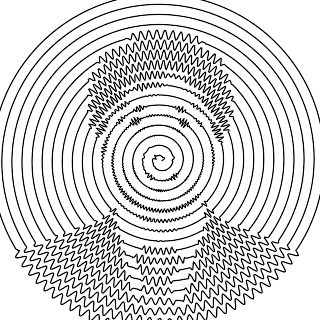I’m grad I’ve finally proven that I can live without Mathematica!